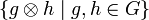# Tensor square of a group

## Definition

### Arbitrary group

Suppose$G$ is a (not necessarily abelian) group. The tensor square of$G$ (sometimes termed the non-abelian tensor square) refers to the tensor product of groups$G \otimes G$ where we take both the actions of$G$ on each other to be the action by conjugation.

Explicitly, it is the quotient of the free group on all formal symbols$\{ g \otimes h \mid g,h \in G \}$ by the following relations:

•$(g_1g_2) \otimes h = (g_1g_2g_1^{-1} \otimes g_1hg_1^{-1})(g_1 \otimes h)$, one such relation for all$g_1,g_2,h \in G$
•$g \otimes (h_1h_2) = (g \otimes h_1)(h_1gh_1^{-1} \otimes h_1h_2h_1^{-1})$, one such relation for all$g,h_1,h_2 \in G$

### Abelian group

For$G$ an abelian group, the tensor square of$G$ is the same as its tensor square as a group. However, it can now also be thought of as follows: it is the tensor product of$G$ with itself in the sense of tensor product of abelian groups.

## Particular cases

### Particular groups

Group Order Tensor square Kernel of homomorphism from tensor square to exterior square Exterior square (as an abstract group) Kernel of homomorphism from tensor square to derived subgroup Schur multiplier (kernel of homomorphism from exterior square to derived subgroup) as a subgroup of exterior square Derived subgroup in the whole group
trivial group 1 trivial group trivial subgroup in trivial group trivial group trivial subgroup in trivial group trivial subgroup in trivial group trivial group
cyclic group:Z2 2 cyclic group:Z2 cyclic group:Z2 as a subgroup of itself trivial group cyclic group:Z2 as a subgroup of itself trivial subgroup of trivial group trivial group
cyclic group:Z3 3 cyclic group:Z3 cyclic group:Z3 as a subgroup of itself trivial group cyclic group:Z3 as a subgroup of itself trivial subgroup of trivial group trivial group
cyclic group:Z4 4 cyclic group:Z4 cyclic group:Z4 as a subgroup of itself trivial group cyclic group:Z4 as a subgroup of itself trivial subgroup of trivial group trivial group
Klein four-group 4 elementary abelian group:E16 elementary abelian group:E8 as a subgroup of elementary abelian group:E16 cyclic group:Z2 elementary abelian group:E16 as a subgroup of itself cyclic group:Z2 as a subgroup of itself trivial group
cyclic group:Z5 5 cyclic group:Z5 cyclic group:Z5 as a subgroup of itself trivial group cyclic group:Z5 as a subgroup of itself trivial subgroup of trivial group trivial group
cyclic group:Z6 6 cyclic group:Z6 cyclic group:Z6 as a subgroup of itself trivial group cyclic group:Z6 as a subgroup of itself trivial subgroup of trivial group trivial group